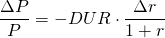# Interest rate and bond value

As explained in the previous article, duration measures a bond’s interest rate risk. We will now explain the inverse relationship between interest rates and a bond’s present value. Additionally, we show how duration can be used both as a risk metric and as an approximation of a bond’s value changes in interest rates.

## Bond value and interest rate changes

Suppose we have a bond with a 5 year maturity, a principal of 1000, a coupon rate of 5% a year. The initial market discount rate is 6.5%. Based on our previous section on fixed rate bond valuation we can calculate the value of the bond to be equal to 937.66. Suppose the market discount rate drops by 1 percentage point. In that case, using a discount rate of 5.5% instead of 6.5%, we obtain a bond value of 978.65. Clearly, the value of our bond has increased by 40.98 or 4.37%.

## Asymmetry in bond value changes

A very special feature of bonds is that changes in interest rates and the resulting changes in the bonds’ value are not linear. As a result, bonds’ changes in value are asymmetric for declines and increases in interest rates. In the previous example, if the market interest rate increase by 1% point, we find that the bond is now worth 898.85. This equals a decrease in value of 38.81 or 4.14% which is lower than 40.98 or 4.37% when interest rates decreased by 1% point.

## Bond value and duration

The new bond value after an interest rate change can be approximated based on the following formula which calculated the approximated percentage change in the bond value. However, this only a good approximation for small interest rate changes. When large changes are considered, adjustments for convexity are required.For our previous bond, the approximated change equals 4.25% or 39.87. These are fairly close to 4.37% and 4.14% or 40.98 and 38.81. Moreover, it becomes apparent that the duration is a linear approximation, both increase and decrease are equal based on the duration.

## Summary

The interest rate and bond value have an inverse relationship. The duration can be used to approximate the future value of the bond when interest rate changes. The bond value change is asymmetric, the increase in value when interest rate falls is larger than the bond value decline when interest rate increases (to the same extent).

### Interest rate and bond value

Need to have more insights? Download our free excel file: interest rate and bond value.